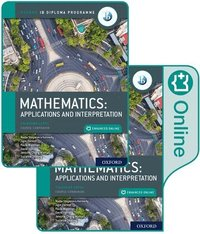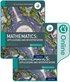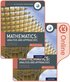Fler böcker inom
Format
Mixed media product
Språk
Engelska
Antal sidor
672
Utgivningsdatum
2019-02-21
Förlag
OUP Oxford
Medarbetare
Waldman, Paula / Chang Wathall, Jennifer / Doering, Suzanne / Harris, David / Stoyanova Kennedy, Nadia
Illustratör/Fotograf
Colour
Illustrationer
Colour
Dimensioner
254 x 196 x 23 mm
Vikt
1952 g
Antal komponenter
1
ISBN
9780198426981

### Du kanske gillar# Oxford IB Diploma Programme: IB Mathematics: applications and interpretation, Standard Level, Print and Enhanced Online Course Book Pack

Mixed media product Engelska, 2019-02-21
749
Skickas inom 10-15 vardagar.
Fri frakt inom Sverige för privatpersoner.
Featuring a wealth of digital content, this concept-based Print and Enhanced Online Course Book Pack has been developed in cooperation with the IB to provide the most comprehensive support for the new DP Mathematics: applications and interpretation SL syllabus, for first teaching in September 2019.

## Passar bra ihop

1.2. +
3.De som köpt den här boken har ofta också köpt Oxford IB Diploma Programme: IB Mathematics: an... av Paul La Rondie (mixed media product).

## Övrig information

Jane Forrest, Paula Waldman, Jennifer Chang Wathall, Suzanne Doering, David Harris, Nadia Stoyanova Kennedy

## Innehållsförteckning

Measuring space: accuracy and 2D geometry 1.1: Measurements and estimates 1.2: Recording measurements, significant digits and rounding 1.3: Measurements: exact or approximate? 1.4: Speaking scientifically 1.5: Trigonometry of right-angled triangles and indirect measurements 1.6: Angles of elevation and depression Representing space: non-right angled trigonometry and volumes 2.1: Trigonometry of non-right triangles 2.2: Area of triangle formula. Applications of right and non-right angled trigonometry 2.3: Geometry: solids, surface area and volume Representing and describing data: descriptive statistics 3.1: Collecting and organising univariate data 3.2: Sampling techniques 3.3: Presentation of data 3.4: Bivariate data Dividing up space: coordinate geometry, lines, Voronoi diagrams 4.1: Coordinates, distance and midpoint formula in 2D and 3D 4.2: Gradient of lines and its applications 4.3: Equations of straight lines; different forms of equations 4.4: Parallel and perpendicular lines 4.5: Voronoi diagrams and toxic waste problem Modelling constant rates of change: linear functions 5.1: Functions 5.2: Linear Models 5.3: Arithmetic Sequences 5.4: Modelling Modelling relationships: linear correlation of bivariate data 6.1: Measuring correlation 6.2: The line of best fit 6.3: Interpreting the regression line Quantifying uncertainty: probability, binomial and normal distributions 7.1: Theoretical and experimental probability 7.2: Representing combined probabilities with diagrams 7.3: Representing combined probabilities with diagrams and formulae 7.4: Complete, concise and consistent representations 7.5: Modelling random behaviour: random variables and probability distributions 7.6: Modelling the number of successes in a fixed number of trials 7.7: Modelling measurements that are distributed randomly Testing for validity: Spearman's, hypothesis testing and x2 test for independence 8.1: Spearman's rank correlation coefficient 8.2: chi2 test for independence 8.3: chi2 goodness of fit test 8.4: The t-test Modelling relationships with functions: power functions 9.1: Quadratic models 9.2: Problems involving quadratics 9.3: Cubic models, power functions and direct and inverse variation 9.4: Optimisation Modelling rates of change: exponential and logarithmic functions 10.1: Geometric sequences and series 10.2: Compound interest, annuities, amortization 10.3: Exponential models 10.4: Exponential equations and logarithms Modelling periodic phenomena: trigonometric functions 11.1: An introduction to periodic functions 11.2: An infinity of sinusoidal functions 11.3: A world of sinusoidal models Analyzing rates of change: differential calculus 12.1: Limits and derivatives 12.2: Equation of tangent and normal and increasing and decreasing functions 12.3: Maximum and minimum points and optimisation Approximating irregular spaces: integration 13.1: Finding areas 13.2: Integration: the reverse processes of differentiation Exploration